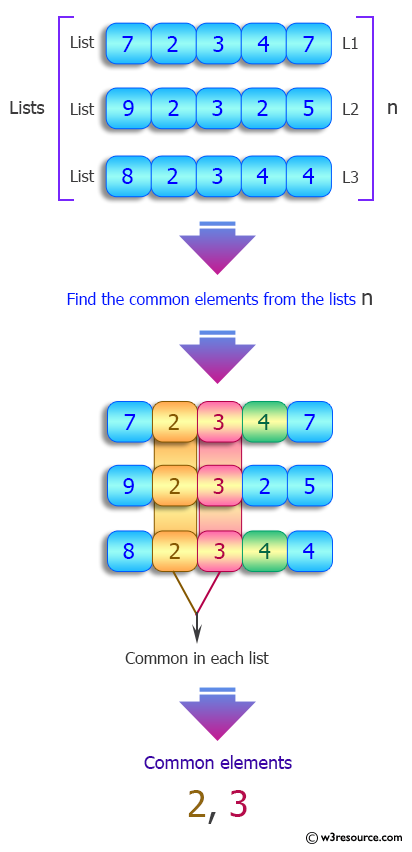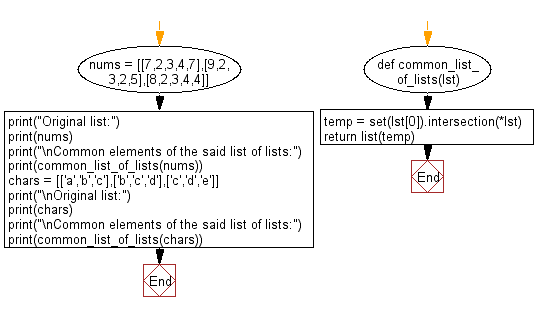﻿ Python: Common elements in a given list of lists - w3resource# Python: Common elements in a given list of lists

## Python List: Exercise - 177 with Solution

Write a Python program to find common elements in a given list of lists.

Sample Solution:

Python Code:

``````def common_list_of_lists(lst):
temp = set(lst).intersection(*lst)
return list(temp)

nums = [[7,2,3,4,7],[9,2,3,2,5],[8,2,3,4,4]]
print("Original list:")
print(nums)
print("\nCommon elements of the said list of lists:")
print(common_list_of_lists(nums))
chars = [['a','b','c'],['b','c','d'],['c','d','e']]
print("\nOriginal list:")
print(chars)
print("\nCommon elements of the said list of lists:")
print(common_list_of_lists(chars))
```
```

Sample Output:

```Original list:
[[7, 2, 3, 4, 7], [9, 2, 3, 2, 5], [8, 2, 3, 4, 4]]

Common elements of the said list of lists:
[2, 3]

Original list:
[['a', 'b', 'c'], ['b', 'c', 'd'], ['c', 'd', 'e']]

Common elements of the said list of lists:
['c']
```

Pictorial Presentation:Flowchart:## Visualize Python code execution:

The following tool visualize what the computer is doing step-by-step as it executes the said program:

Python Code Editor:

Have another way to solve this solution? Contribute your code (and comments) through Disqus.

What is the difficulty level of this exercise?

Test your Python skills with w3resource's quiz

﻿

## Python: Tips of the Day

Floor Division:

When we speak of division we normally mean (/) float division operator, this will give a precise result in float format with decimals.

For a rounded integer result there is (//) floor division operator in Python. Floor division will only give integer results that are round numbers.

```print(1000 // 300)
print(1000 / 300)```

Output:

```3
3.3333333333333335```